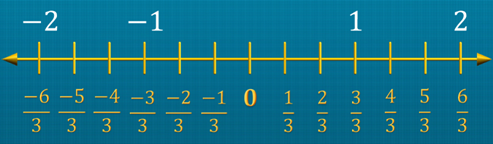Notes On Introduction to Rational Numbers - CBSE Class 7 Maths
All numbers, including whole numbers, integers, fractions and decimal numbers, can be written in the form. Rational number A rational number is a number that can be written in the form p/q, where p and q are integers and q ≠ 0.  The denominator of a rational number can never be zero.  e.g. $\frac{\text{9}}{\text{11}}$, $\frac{\text{5}}{\text{8}}$, $\frac{\text{7}}{\text{12}}$ A rational number is positive if its numerator and denominator are both either positive integers or negative integers. e.g. $\frac{\text{2}}{\text{5}}$, $\frac{\text{3}}{\text{4}}$, $\frac{\text{-7}}{\text{-10}}$, $\frac{\text{-5}}{\text{-11}}$ If either the numerator or the denominator of a rational number is a negative integer, then it is a negative rational number.  e.g. $\frac{\text{-2}}{\text{5}}$, $\frac{\text{3}}{\text{-4}}$, $\frac{\text{7}}{\text{-10}}$, $\frac{\text{5}}{\text{-11}}$ Representation of rational numbers on the number lineThe rational number zero is neither negative nor positive. Positive rational numbers are represented to the right of zero on the number line. Negative rational numbers are represented to the left of zero on the number line. A rational number obtained by multiplying or dividing both the numerator and the denominator of a rational number by the same non-zero integer, is said to be the equivalent form of the given rational number. Rational numbers in Standard form A rational number is said to be in its standard form if its numerator and denominator have no common factor other than 1, and its denominator is a positive integer.To reduce a rational number to its standard form, divide its numerator and denominator by their highest common factor (HCF). To find the standard form of a rational number with a negative integer as the denominator, divide its numerator and denominator by their HCF with a minus sign.

#### Summary

All numbers, including whole numbers, integers, fractions and decimal numbers, can be written in the form. Rational number A rational number is a number that can be written in the form p/q, where p and q are integers and q ≠ 0.  The denominator of a rational number can never be zero.  e.g. $\frac{\text{9}}{\text{11}}$, $\frac{\text{5}}{\text{8}}$, $\frac{\text{7}}{\text{12}}$ A rational number is positive if its numerator and denominator are both either positive integers or negative integers. e.g. $\frac{\text{2}}{\text{5}}$, $\frac{\text{3}}{\text{4}}$, $\frac{\text{-7}}{\text{-10}}$, $\frac{\text{-5}}{\text{-11}}$ If either the numerator or the denominator of a rational number is a negative integer, then it is a negative rational number.  e.g. $\frac{\text{-2}}{\text{5}}$, $\frac{\text{3}}{\text{-4}}$, $\frac{\text{7}}{\text{-10}}$, $\frac{\text{5}}{\text{-11}}$ Representation of rational numbers on the number lineThe rational number zero is neither negative nor positive. Positive rational numbers are represented to the right of zero on the number line. Negative rational numbers are represented to the left of zero on the number line. A rational number obtained by multiplying or dividing both the numerator and the denominator of a rational number by the same non-zero integer, is said to be the equivalent form of the given rational number. Rational numbers in Standard form A rational number is said to be in its standard form if its numerator and denominator have no common factor other than 1, and its denominator is a positive integer.To reduce a rational number to its standard form, divide its numerator and denominator by their highest common factor (HCF). To find the standard form of a rational number with a negative integer as the denominator, divide its numerator and denominator by their HCF with a minus sign.

Previous